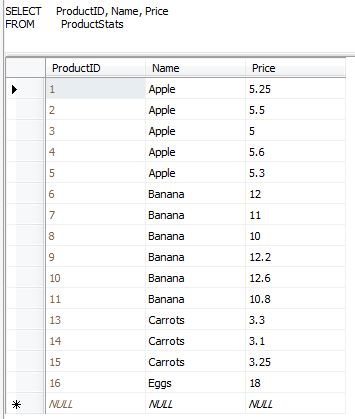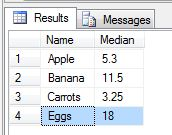# Median Calculation using TSQL (no cursors)

By | October 13, 2010

Before we start lets define what a Median is first, in laymans term the Median is the “middle number” in a sorted list of numbers.

So in a sample like

### 3, 4, 5, 8, 10, 11, 100

The middle number which is the median as well is number 8 as you have 3 numbers in before and after number 8, now  what if there are two numbers in the middle and that will happen if there is an even amount of number, in that case we need to find the middle two numbers then get their average or add them (the 2 middle numbers) together and divide them by 2 so on the example earlier lets try to add another number

### 3, 4, 5, 8, 10, 11, 100, 101

So here the middle number is 8 and 10 and their average is 9 so thats the median number.

Now you know you know what median is, now we try to apply that in SQL, there are a lot of solution but mostly  it uses cursors or complex TSQL queries as its not directly supported by TSQL, and here are the only grouping functions available, isn’t it good to add the median function in the next versions of SQL

thanks to CTE or common table expressions things got easiser.

So lets get started lets say you have this table and you want to calculate the median price for each productNow to view it easily you can sort it by Price and get the middle numbersNow to achieve that on TSQL, here are the codes

```With
MedianResults
as
(
Select
Name,Price ,
Row_Number() OVER(Partition by Name Order by Price) as A,
Row_Number() OVER(Partition by Name Order by Price desc) as B
from ProductStats

)
Select Name, Avg(Price) as Median
From MedianResults
Where Abs(A-B)<=1
Group by Name```

Doing that, the results will yeildUPDATE!!!

One of my article readers at code project found a bug on the above post and it happens if you have values that are “tied”, the ROW_NUMBER result is not “aligned” as you might want, and it produces an incorrect value.  As he suggested I used a set with tied numbers such as

### 3, 4, 5, 8, 101, 101, 101, 101

and in that set it sould output 54.5 but it did not and isntead its outputting 8 instead as the ROW_NUMBERS becomes unaligned when you use similar value digits.  So his solution was this :

```WITH
ResultA
AS
(SELECT	Name, Price, ROW_NUMBER() OVER ( PARTITION BY Name ORDER BY Price ) AS A FROM  ProductStats),
ResultB
AS
(SELECT	Name, Price, A, ROW_NUMBER() OVER ( PARTITION BY Name ORDER BY Price DESC, A DESC ) AS B FROM ResultA)
SELECT
Name,
Avg(Price) as Median
FROM ResultB
WHERE  Abs(A - B) <= 1
GROUP BY Name```

and I totally agree!

## One thought on “Median Calculation using TSQL (no cursors)”

This site uses Akismet to reduce spam. Learn how your comment data is processed.